Electromagnetic Waves in a Cubical Cavity

Electromagnetic standing waves in a cavity at equilibrium with its surroundings cannot take just any path. They must satisfy the wave equation in three dimensions: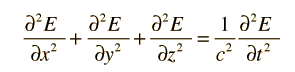The solution to the wave equation must give zero amplitude at the walls, since a non-zero value would dissipate energy and violate our supposition of equilibrium. To form a standing wave, the reflection path around the cavity must produce a closed path. The boundary conditions can be met with a solution of the form: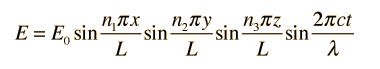Substituting this solution into the wave equation above gives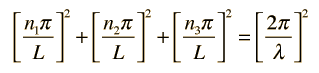which simplifies toDevelopment of the Rayleigh-Jeans Law

Index

Rayleigh- Jeans references

 HyperPhysics***** Quantum Physics R Nave
Go Back

How many modes in the cavity?

From the standing wave solution to the wave equation we get the condition.The Rayleigh scheme for counting modes. After Richtmyer, et al. We need to evaluate the number of modes which can meet this condition, which amounts to counting all the possible combinations of the integer n values. An approximation can be made by treating the number of combinations as the volume of a three-dimensional grid of the values of n, an "n-space". Using the relationship for the volume of a sphere, with the n values specifying the coordinates along three "n" axes, gives.

This has a couple of problems, however. In using a sphere, we have used both positive and negative values of n, whereas the wave equation solution uses only positive definite values. Therefore we must take 1/8 th of the volume above. Another technical problem is that you can have waves polarized in two perpendicular planes, so we must multiply by two to account for that. Then the volume can be taken to be a measure of the number of modes, becoming a very good approximation when the size of the cavity is much greater than the wavelength as in the case of electromagnetic waves in finite cavity. Using the relation obtained for the values of n, this becomes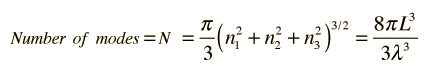Development of the Rayleigh-Jeans Law

Index

Rayleigh- Jeans references

Richtmyer, et al.
Ch. 5

 HyperPhysics***** Quantum Physics R Nave
Go Back

How many modes per unit wavelength?

Having developed an expression for the number of standing wave modes in a cavity, we would like to know the distribution with wavelength. This may be obtained by taking the derivative of the number of modes with respect to wavelength.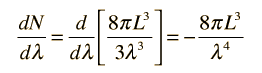The negative sign here reveals that the number of modes decreases with increasing wavelength. Now to get the number of modes per unit volume per unit wavelength, we can simply divide by the volume of the cubical cavity.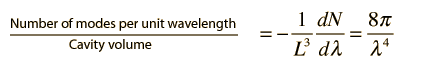Note that this does not involve approximating a sphere with a cube! The sphere we used in calculating the number of modes was a sphere in "n-space", allowing us to count the number of possible modes. Also, the use of a cubical cavity for the calculation just allows us to reduce the geometrical complexity of the development, but the final result obtained is independent of cavity geometry.

Development of the Rayleigh-Jeans Law

Index

Rayleigh- Jeans references

 HyperPhysics***** Quantum Physics R Nave
Go Back

How much energy per unit volume?

Assigning energy to the electromagnetic standing waves in a cavity draws on the principle of equipartition of energy. Each standing wave mode will have average energy kT where k is Boltzmann's constant and T the temperature in Kelvins. Letting u represent the energy density:This is an important relationship in classical electromagnetic cavity theory. It can also be expressed in terms of the frequency ν by making use of the chain rule and the wave relationship: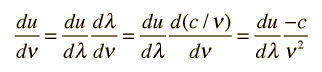The minus sign here just reminds us that a decrease with wavelength implies an increase with increasing frequency. The magnitude of the energy density dependence on frequency is given by: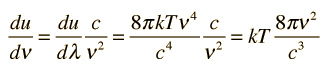Note that this is the classical result which was used in the Rayleigh-Jeans Law, but led to the ultraviolet catastrophe. It produces good agreement in the low frequency limit, but for higher frequencies the Planck radiation formula must be used.

Development of the Rayleigh-Jeans Law

Index

Rayleigh- Jeans references

 HyperPhysics***** Quantum Physics R Nave
Go Back

Radiated Energy as a Function of Wavelength

If we consider energy radiated perpendicular to a small increment of area, then it must be noted that half of the energy density in the waves is going toward the walls and half is coming out if the system is in thermal equilibrium. Evaluating the power seen at a given observation point requires a consideration of the geometry: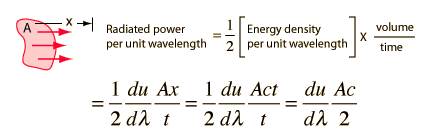but at an angle θ, the effective area will be Acosθ and the effective speed will be c cosθ, so the radiated energy will be reduced toFor a given observation point near a radiating surface, the power will be the average power from all directions, and the average gives another factor of 1/2.

Having averaged over all angles, the calculated radiated power per unit wavelength is finally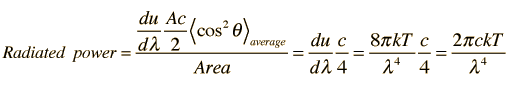More detailed development of the c/4 factor

This is the Rayleigh-Jeans formula. The fact that it failed to predict the spectral distribution from hot objects was one of the major unresolved issues in physics at the beginning of the 20th century.
To express this in terms of frequency, an application of the chain rule as was done above with the energy density yields a radiated power per unit frequency:Development of the Rayleigh-Jeans Law

Index

Rayleigh- Jeans references